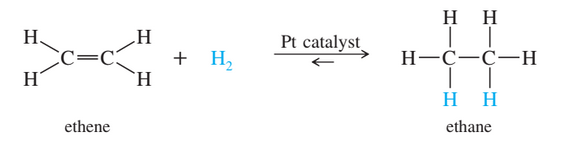×
Get Full Access to Organic Chemistry - 8 Edition - Chapter 4 - Problem 7p
Get Full Access to Organic Chemistry - 8 Edition - Chapter 4 - Problem 7p

×

# When ethene is mixed with hydrogen in the presence of aISBN: 9780321768414 33

## Solution for problem 7P Chapter 4

Organic Chemistry | 8th Edition

• Textbook Solutions
• 2901 Step-by-step solutions solved by professors and subject experts
• Get 24/7 help from StudySoup virtual teaching assistantsOrganic Chemistry | 8th Edition

4 5 1 386 Reviews
23
4
Problem 7P

When ethene is mixed with hydrogen in the presence of a platinum catalyst, hydrogen adds across the double bond to form ethane. At room temperature, the reaction goes to completion. Predict the signs of $$\Delta H^{\circ} \text { and } \Delta S^{\circ}$$  for this reaction. Explain these signs in terms of bonding and freedom of motion.Equation Transcription:Text Transcription:

\Delta H^\circ  and \Delta S^\circ

H_2

Step-by-Step Solution:

Solution 7P

Step 1

Ethane undergoes catalytic reduction in presence of hydrogento form ethane.

The reaction is as follows:Step 2 of 3

Step 3 of 3

## Discover and learn what students are asking

Calculus: Early Transcendental Functions : Antiderivatives and Indefinite Integration
?In Exercises 7–10, complete the table to find the indefinite integral. Original Integral Rewrite

Calculus: Early Transcendental Functions : Inverse Trigonometric Functions: Integration
?In Exercises 1-20, find the indefinite integral. $$\int \frac{1}{x \sqrt{4 x^{2}-1}} d x$$

Calculus: Early Transcendental Functions : Inverse Trigonometric Functions: Integration
?In Exercises 1-20, find the indefinite integral. $$\int \frac{12}{1+9 x^{2}} d x$$

Statistics: Informed Decisions Using Data : Organizing Qualitative Data
?In a relative frequency distribution, what should the relative frequencies add up to?

Unlock Textbook Solution